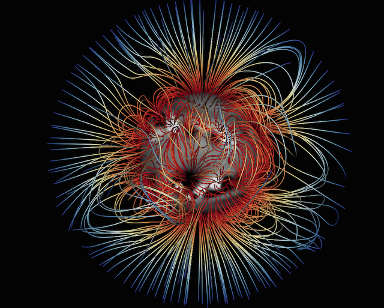## Magnetic Equilibria

### Description

The Sun's atmosphere is typical of astrophysical plasmas in that its shape and dynamics are controlled by the Sun's magnetic field. However, the very low particle density prevents us from measuring this magnetic field directly, so mathematical models are required to extrapolate this magnetic field from observations on the Sun's surface.

This project will explore the equilibrium solutions used to model such magnetic fields. The simplest model involves solving Laplace's equation, but more sophisticated models require so-called force-free fields. Nonlinearity of the governing equation makes such equilibria a continuing mathematical and/or computational challenge.Numerical force-free field model of the Sun's magnetic field.

We will start by reviewing the equations that govern the magnetic field, and looking at basic solutions. Then there are many possibilities for individual students to specialise based on current research. For example, the numerically inclined could look into extrapolation techniques for the Sun's magnetic field (using Python). The theoretically inclined could look at the variational principles obeyed by magnetic equilibria, or at "magneto-hydrostatic" solutions where pressure gradients and gravity are also accounted for.

### Prerequisites

Analysis in Many Variables II (or Mathematical Methods in Physics) is essential. Special Relativity & Electromagnetism II would be useful. Continuum Mechanics III and/or Partial Differential Equations III would be useful to take alongside this project.

### Resources

For recent overviews of magnetic equilibria in the solar corona, see the Living Reviews by Wiegelmann and Sakurai, or Section 3 of Mackay and Yeates.

We will start by working through Chapter 13 of Sturrock, Plasma physics. Other useful books include Marsh, Force-free magnetic fields: solutions, topology and applications, Choudhuri, The physics of fluids and plasmas, and Priest, Magnetohydrodynamics of the Sun.

email: Anthony Yeates